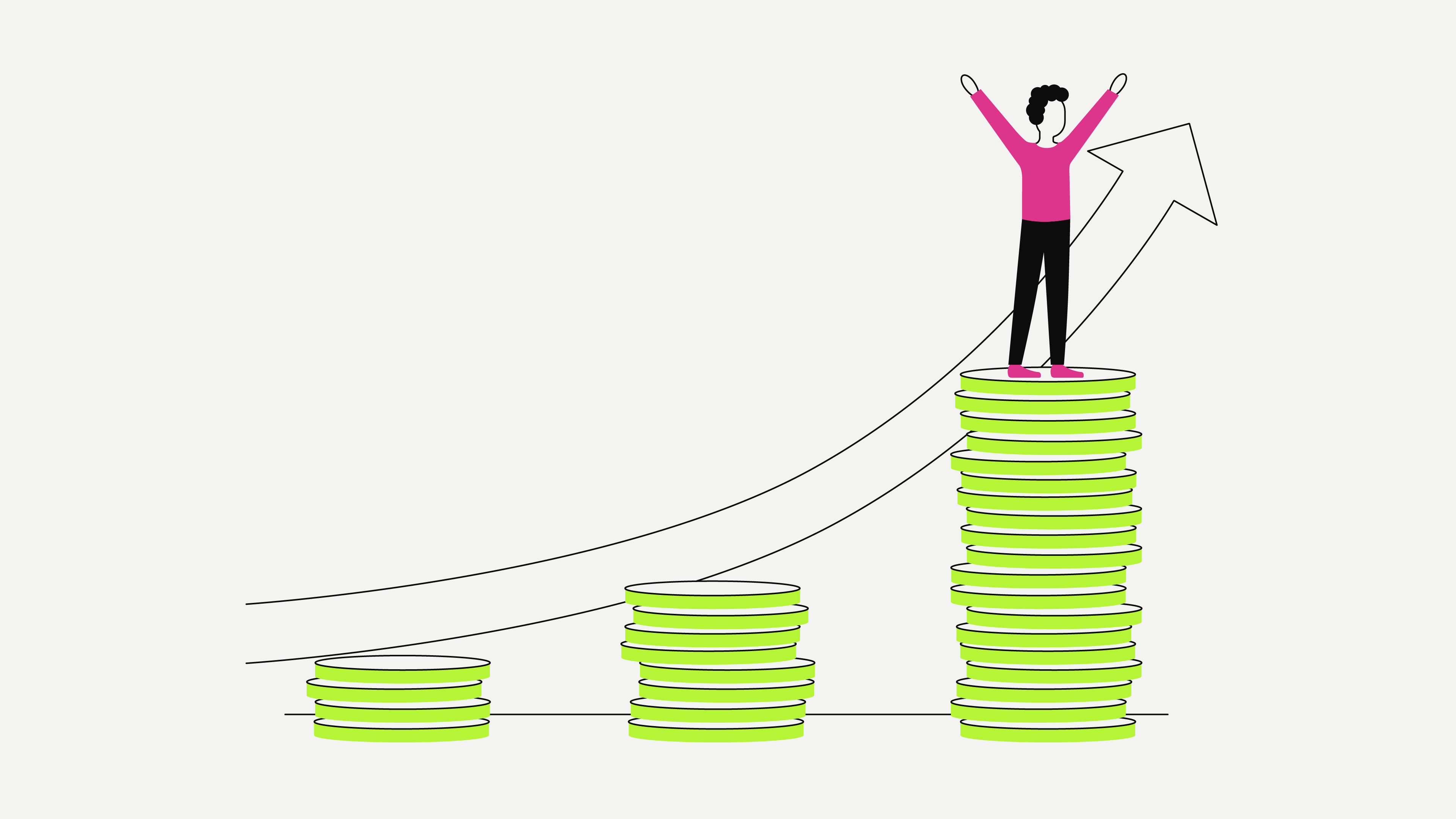Personal Finance
Lesson 8
3 min

# What is interest?

## In the context of investing, interest is what the investor earns for placing their money in an investment or a project. In the context of loans, it is the amount a debtor pays for borrowing money.

• Interest is calculated based on the original amount of money that is invested.

• An interest rate is the percentage of how much and over what period of time the bank wants to charge you for giving you a loan, or what the bank wants to give you for depositing your money in a savings account with them.

• If you invest your money in interest-generating assets, you will earn interest on that amount, and at a later stage, compound interest.

• Compound interest is created from interest that is already credited to an account - it is interest on top of already existing interest.

## The history of interest

According to historical sources, committing resources to achieve a return was first documented as early as 1700 BC in the Code of Hammurabi. Hammurabi, ruler of Babylonia, had drawn up a code that was to be applied to his entire empire. The code was carved into a stone stele, and today this stele is on display at the Louvre in Paris, France. The Code of Hammurabi states that “A merchant may collect interest of thirty-three and one-third percent on a loan of grain, and twenty percent interest may be charged on a loan of silver.”

## How is interest calculated?

In the context of debt, interest is an amount of money that the debtor (the person borrowing the money) pays the creditor (the person or institution lending the money) in return for lending the amount borrowed (the capital) for a certain amount of time.

In the context of investing, interest is the percentage amount an investor earns for placing their money into a certain project or investment.

Whether for loans, savings accounts, or investing, the amount of interest is calculated as a percentage of the loan balance or the deposit balance. The interest rate is pre-determined or calculated by the creditor using this set percentage.

This is the formula commonly used for the most basic calculation for simple interest:

Simple Interest = P (Principal amount, the original amount of money) x R (Rate of interest) x Time (Duration of loan/investment period)

Let’s assume our investor deposits EUR 15,000 into a savings account that earns 2 percent (0.02) interest for five years. The interest the investor would earn over five years is: 15,000 times 0.02 times 5, which amounts to EUR 1,500.

Remember that investing means putting money into something in the hopes of achieving financial gain from it. In the context of asset generation, interest is the amount of money an investor receives for placing their money in a savings account. For this, they are paid interest.

## What is compound interest?

Investors thinking about the long term don’t rely on simple interest; instead, they use the power of compound interest to increase their wealth. They earn interest over the long term, called compounding, which is the equivalent of “magnifying”. This is achieved with discipline and perseverance.

This article does not constitute investment advice, nor is it an offer or invitation to purchase any digital assets.